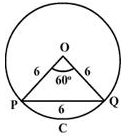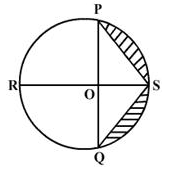Courses

# Test: Areas Of Sectors And Segments

## 20 Questions MCQ Test Mathematics (Maths) Class 10 | Test: Areas Of Sectors And Segments

Description
This mock test of Test: Areas Of Sectors And Segments for Class 10 helps you for every Class 10 entrance exam. This contains 20 Multiple Choice Questions for Class 10 Test: Areas Of Sectors And Segments (mcq) to study with solutions a complete question bank. The solved questions answers in this Test: Areas Of Sectors And Segments quiz give you a good mix of easy questions and tough questions. Class 10 students definitely take this Test: Areas Of Sectors And Segments exercise for a better result in the exam. You can find other Test: Areas Of Sectors And Segments extra questions, long questions & short questions for Class 10 on EduRev as well by searching above.
QUESTION: 1

Solution:
QUESTION: 2

Solution:
QUESTION: 3

### PQ is a chord of a circle with centre O and radius 6cm. PQ is of length 6 cm and divides the circle into two segments. The area of the minor segment isSolution:
QUESTION: 4

The length of a minute hand of a wall clock is 7 cm. What is the area swept by it in 30 minutes​

Solution:
QUESTION: 5

In the given figure PQ and RS are the perpendicular diameters of the circle whose centre is O and radius = 14 cm. the area of the shaded region isSolution:
QUESTION: 6

A pendulum swings through an angle of 300 and describes an arc 8.8cm in length. The length of the pendulum is​

Solution:
QUESTION: 7

Three horses are tethered with 7 meter long ropes at the three corners of a triangular field having sides 20 m, 34 m and 42 m. The area of the plot which can be grazed by the horses is​

Solution:
QUESTION: 8

Area of a quadrant of circle whose circumference is 22 cm is : (= 22/7)​

Solution:
QUESTION: 9

The length of an arc that subtends an angle of 24° at the centre of a circle with 5 cm radius is

Solution:
QUESTION: 10

The length of a minute hand of a wall clock is 7 cm. What is the area swept by it is 30 minutes?​

Solution:

Explanation:-

The area covered by the minute hand in 60 minutes is equal to area of the circle of radius 7 cm

The area covered by minute hand in 30 minutes will be half of the area of the circle.

We know, area of the circle = π r^2

Half of the area of the circle = ½ π r^2

Here, π = 22/7  and r = 7

=>  ½ *22/7 * (7) *(7)

=>  11 * 7

=> 77 cm square

QUESTION: 11

The angle of the major sector and the corresponding minor sector of a circle are​

Solution:
QUESTION: 12

The area of a sector of a circle of radius 5 cm is 5π cm2. The angle contained by the sector will be​

Solution:
QUESTION: 13

If the ratio of the arc AB to the circumference of a circle is 1:3,then measure of the angle subtended by the arc at the centre is​

Solution:
QUESTION: 14

To warn ships of underwater rocks, a light house spreads a red colored light over a sector of angle 80° to a distance of 16.5 km. The area of the sea over which the ships are warned is​

Solution:
QUESTION: 15

The minute hand of a clock is 10 cm long. The area of the face of the clock described by the minute hand between 8 A.M and 8.25 A.M is​

Solution:
QUESTION: 16

The perimeter of a certain sector of a circle of radius 6.5 cm is 31cm. The area of the sector will be​

Solution:
QUESTION: 17

A circular disc of radius 6 cm is divided into three sectors with central angles 90°, 120° and 150°. The ratio of the areas of the three sectors is

Solution:
QUESTION: 18

If the area of a sector POK is 100π sq.units and ∠POK = 49°, then find the radius of sector

Solution:
QUESTION: 19

What is the area of a regular hexagon inscribed in a circle of radius r units?

Solution:
QUESTION: 20

Area of a sector of a circle of radius 36 cm is 54π cm2. The length of the corresponding arc of the sector is:​

Solution: